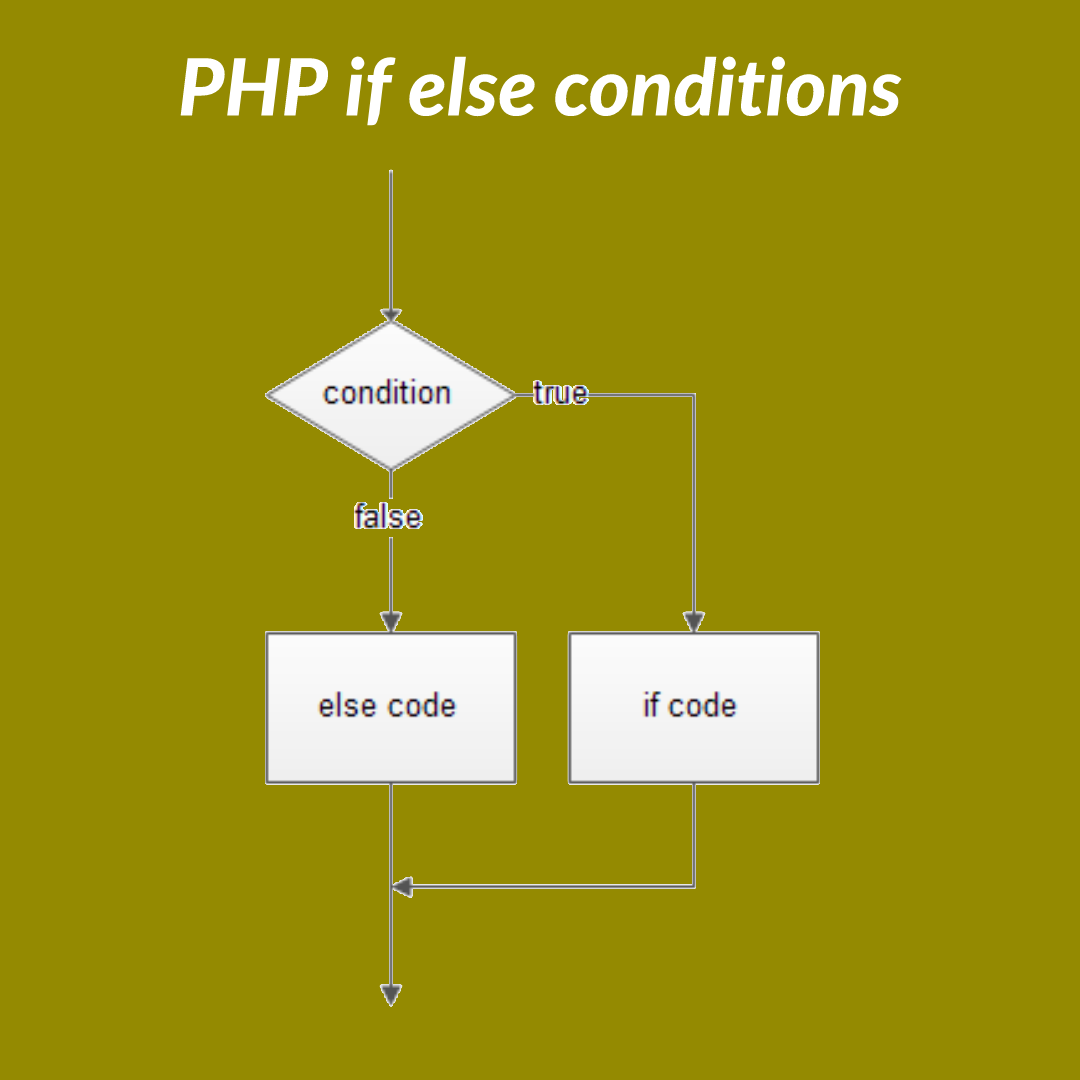# PHP If Else ConditionPHP if else condition is the most important part and also has too many variations, so first we are going to discuss basic of PHP if else condition, and then will check nested if-else, writing style of if-else condition, etc.

## Basic of PHP if else condition

an if condition is mainly written by if keyword and then inside first brasses the condition, and then if there is no defined scope by Carly brasses or: and end if then where it will get the first semicolon (;) after the if the condition it will be the if conditions scope.

Example:

<?php
\$i = 1;

if(\$i==0)
echo ‘value of \$i = ‘ . \$i; // after if condition where it will get the first semicolon it will be
// the end of the scope of the if condition
// so it will not print anything

if(\$i==0);
echo ‘value of \$i = ‘ . \$i; // after if condition where it will get the first semicolon it will be
// the end of the scope of the if condition
// so it will print anything value of \$i = 1

?>

## PHP Writing Style

There is several style to write php if else condition and below we are going to see example of them.

Simple One Line PHP if else condition

for next line if condition validation , the writing style is first if then condition then , on condition applied what to do line and at last semicolon

Example:

<?php
\$i = 1;
if(\$i==0)
echo ‘value of \$i = ‘ . \$i;
?>

## Multi Line scope if condition

To validate multi line or execute multiple lines if the condition satisfy then after if condition just use Carly brasses and write the codes inside the Carly brasses.

Example:

<?php
\$i = 1;
if(\$i==1){
echo ‘value of \$i = ‘ . \$i;
echo ‘The if condition satisfyed’;
}
?>

## Multi Line scope if else condition

To validate multi line or execute multiple lines if the condition satisfy then after if condition just use Carly brasses and write the codes inside the Carly brasses. and if not satisfied then use else part and then inside the Carly brasses write the codes.

Example:

<?php
\$i = 1;
if(\$i==0){
echo ‘value of \$i = ‘ . \$i;
echo ‘The if condition satisfyed’;
}else{
echo ‘value of \$i = ‘ . \$i;
echo ‘The if condition not satisfyed’;
}

or

if(\$i==0){
echo ‘value of \$i = ‘ . \$i;
echo ‘The if condition satisfyed’;
}else
echo ‘The if condition not satisfied’;

?>

## Multi Line scope if condition by not using Carly brasses

To validate multi line or execute multiple lines if the condition satisfy then after if condition just use : and end if and write the codes inside the Carly brasses.

Example:

<?php
\$i = 1;
if(\$i==1):
echo ‘value of \$i = ‘ . \$i;
echo ‘The if condition satisfyed’;
endif;
?>

## Multi Line scope if else condition by not using Carly brasses

To validate multi line or execute multiple lines if the condition satisfy then after if condition just use : and end if and write the codes inside the Carly brasses. ind if not satisfied then write the code after else.

Example:

<?php
\$i = 1;
if(\$i==0):
echo ‘value of \$i = ‘ . \$i;
echo ‘The if condition satisfyed’;
else:
echo ‘value of \$i = ‘ . \$i;
echo ‘The if condition not satisfyed’;
endif;
?>

## How to use the ternary operator ( ? : ) in PHP as a shorthand for “if / else”.

to use the ternary first write the condition then give question mark and then write the code which shall execute while the condition is satisfied and then give : (colon) and then write the code if the condition not satisfied.

Example:

<?php
\$i = 1;
\$j = 2;
\$a = (\$i==\$j) ? “The if condition satisfyed” : ‘The if condition not satisfyed’;
echo \$a; // it will print The if condition not satisfyed
?>

## PHP Nested if else condition

a nested if else if if case inside if case or else case there is a waterfall model of ef else condition and one example of nested if else condition is given below.

Example:

<?php

\$i = 1;
\$j = 2;
\$k = 2;
\$l = 2;

if (\$i==\$j) {
if (\$i==\$k) {
echo “j = k”;
}else{
echo “j != k”;
}
}else if(\$j == \$k){
if (\$j==\$l):
echo “j = l”;
else if(\$i == \$k):
echo “i = k”;
else:
echo “i != k”;
endif;
}else{
if(\$i==\$l){
\$a = (\$i==\$j) ? “The if condition satisfyed” : ‘The if condition not satisfyed’;
echo \$a;
}
}

?>

you have gone through the post PHP If Else Condition and i hope that it helped you to learn php.